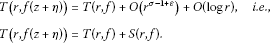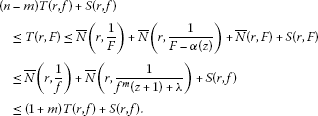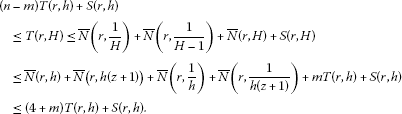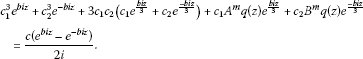Theory and Modern Applications

# Some results on zeros and the uniqueness of one certain type of high difference polynomials

## Abstract

In this paper, we investigate the distribution of the zeros of some high differential-difference polynomials and obtain some uniqueness theorems with regard to it.

MSC:30D35, 34M10.

## 1 Introduction and main results

In this paper, a meromorphic function always means it is meromorphic in the whole complex plane $\mathbb{C}$. We assume that the reader is familiar with the standard notations in the Nevanlinna theory. We use the following standard notations in value distribution theory (see [1, 4, 11, 14]):

$T\left(r,f\right),m\left(r,f\right),N\left(r,f\right),\overline{N}\left(r,f\right),\dots .$

And we denote by $S\left(r,f\right)$ any quantity satisfying

possibly outside of a set E with finite linear measure, not necessarily the same at each occurrence. A meromorphic function $a\left(z\right)$ is said to be a small function with respect to $f\left(z\right)$ if $T\left(r,a\right)=S\left(r,f\right)$. We say that two meromorphic functions $f\left(z\right)$ and $g\left(z\right)$ share the value a IM (ignoring multiplicities) if $f\left(z\right)-a$ and $g\left(z\right)-a$ have the same zeros. If $f\left(z\right)-a$ and $g\left(z\right)-a$ have the same zeros with the same multiplicities, then we say that they share the value a CM (counting multiplicities). A polynomial $Q\left(z,f\right)$ is called a differential-difference polynomial in f if Q is a polynomial in f, its derivatives and shifts with meromorphic coefficients, say $\left\{{a}_{\lambda }|\lambda \in I\right\}$, such that $T\left(r,{a}_{\lambda }\right)=S\left(r,f\right)$ for all $\lambda \in I$. We define the difference operators $\mathrm{\Delta }f=f\left(z+1\right)-f\left(z\right)$ and ${\mathrm{\Delta }}^{n}f={\mathrm{\Delta }}^{n-1}\left(\mathrm{\Delta }f\right)$.

In 2007, Laine and Yang  considered zeros of one certain type of difference polynomials and obtained the following theorem.

Theorem A Let f be a transcendental entire function of finite order and c be a nonzero complex constant. If $n\ge 2$, then ${f}^{n}\left(z\right)f\left(z+c\right)-a$ has infinitely many zeros, where $a\in C\setminus 0$.

Liu  considered the case of general difference products of a meromorphic function and made relevant improvements to Theorem A. Recently, a number of papers [5, 6] focusing on the distribution of zeros of some difference polynomials of differential types emerged. In this paper, we consider the general differential difference cases in some sense and obtain some results as follows.

Theorem 1 Let f be a transcendental entire function of finite order and $\alpha \left(z\right)\not\equiv 0$ be a small function with respect to $f\left(z\right)$. If one of the following conditions holds:

1. (i)

$n\ge k+2$ when $m\le k+1$;

2. (ii)

$n\ge 2k-m+3$ when $m\ge k+2$,

then the differential-difference polynomial ${\left[{f}^{n}\left(z\right){f}^{m}\left(z+1\right)\right]}^{\left(k\right)}-\alpha \left(z\right)$ has infinitely many zeros.

Theorem 2 Let f be a transcendental entire function of finite order and ${\mathrm{\Delta }}^{m}f\not\equiv 0$, and $\alpha \left(z\right)\not\equiv 0$ be a small function with respect to $f\left(z\right)$. If $n\ge k+3$, then the differential-difference polynomial ${\left[{f}^{n}\left(z\right){\mathrm{\Delta }}^{m}f\right]}^{\left(k\right)}-\alpha \left(z\right)$ has infinitely many zeros.

Theorem 3 Let f be a transcendental meromorphic function of finite order, λ be a nonzero constant, and $\alpha \left(z\right)\not\equiv 0$ be a small function with respect to $f\left(z\right)$. If $n\ge 2m+2$, then the differential-difference polynomial ${f}^{n}\left(z\right)\left({f}^{m}\left(z+1\right)+\lambda \right)-\alpha \left(z\right)$ has infinitely many zeros.

Theorem 4 Let f, g be two transcendental entire functions of finite order, λ be a nonzero constant, and $\alpha \left(z\right)\not\equiv 0$ be a small function with respect to $f\left(z\right)$. If $n\ge 3m+5$ and ${f}^{n}\left(z\right)\left({f}^{m}\left(z+1\right)+\lambda \right)$, ${g}^{n}\left(z\right)\left({g}^{m}\left(z+1\right)+\lambda \right)$ share $\alpha \left(z\right)$ CM, then one of the following cases holds:

1. (i)

$f=hg$, where ${h}^{n}={h}^{m}=1$, i.e., if m, n are two prime integers, then $f\equiv g$;

2. (ii)

${f}^{n}\left(z\right)\left({f}^{m}\left(z+1\right)+\lambda \right){g}^{n}\left(z\right)\left({g}^{m}\left(z+1\right)+\lambda \right)={\alpha }^{2}\left(z\right)$.

Recently, Yang and Laine  considered the following interesting differential equation and they proved

Theorem B Let $p\left(z\right)$ be a nonvanishing polynomial and $b,c\in C$ be two nonzero complex constants. If $p\left(z\right)$ is nonconstant, then the differential equation

${f}^{3}\left(z\right)+p\left(z\right){f}^{″}\left(z\right)=csinbz$

admits no transcendental entire solutions. If $p\left(z\right)$ is a nonzero constant, then equation above admits three distinct transcendental entire solutions, provided ${\left(\frac{p{b}^{2}}{27}\right)}^{3}=\frac{{c}^{2}}{4}$.

They also presented some results on difference analogues of the equation in Theorem B and obtained the following theorem.

Theorem C A nonlinear difference equation

${f}^{3}\left(z\right)+q\left(z\right)f\left(z+1\right)=csinbz,$

where $q\left(z\right)$ is a nonconstant polynomial and $b,c\in C$ are two nonzero constants, has no transcendental entire function of finite order. If $q\left(z\right)$ is a nonzero constant, then the difference equation above admits three distinct transcendental entire functions of finite order, provided $b=3n\pi$ and ${q}^{3}={\left(-1\right)}^{n+1}\frac{27{c}^{2}}{4}$ for a nonzero integer n.

In this paper, we also consider a more general class of difference equation related to Theorem B and Theorem C and obtain the following results, which generalize the above related results.

Theorem 5 Let p, q be two polynomials and $m\ge 2$, n be two positive integers. Then the difference equation

${f}^{m}\left(z\right)+q\left(z\right){\mathrm{\Delta }}^{n}f=p\left(z\right)$

has no transcendental entire function of finite order.

Theorem 6 Let $q\left(z\right)$ be a nonconstant polynomial and b, c be two nonzero constants. Then the difference equation

${f}^{3}\left(z\right)+q\left(z\right){\mathrm{\Delta }}^{m}f=csinbz$
(1)

has no transcendental entire function of finite order. If $q\left(z\right)$ is a nonzero constant, and the difference equation above admits a transcendental entire function of finite order, then

$b=\frac{6k\pi }{m}+3\pi +6n\pi$

and

${q}^{3}=\frac{-27{c}^{2}}{4{\left(-{e}^{\frac{2k\pi i}{m}}-1\right)}^{3m}},$

where $n\in N$, $k=0,1,\dots ,m-1$.

## 2 Some lemmas

To prove our results, we need some lemmas as follows.

Lemma 1 (see )

Let $f\left(z\right)$ be a transcendental meromorphic function with finite order. Then

$m\left(r,\frac{f\left(z+c\right)}{f\left(z\right)}\right)=S\left(r,f\right).$

Lemma 2 (see )

Let $f\left(z\right)$ be a transcendental meromorphic function with finite order σ and η be a nonzero complex number, then for each $\epsilon >0$, we haveLemma 3 Let $f\left(z\right)$ be a transcendental entire function with finite order and $F={f}^{n}\left(z\right)×{f}^{m}\left(z+1\right)$. Then

$T\left(r,F\left(z\right)\right)=\left(m+n\right)T\left(r,f\right)+S\left(r,f\right).$

Proof On the one hand, from Lemma 1, Lemma 2, and the standard Valiant-Mohon’ko theorem, we obtain

$\begin{array}{rcl}\left(m+n\right)T\left(r,f\right)+S\left(r,f\right)& =& T\left(r,{f}^{m+n}\left(z\right)\right)=m\left(r,{f}^{m+n}\left(z\right)\right)\\ =& m\left(r,F\frac{{f}^{m}\left(z\right)}{{f}^{m}\left(z+1\right)}\right)\\ \le & m\left(r,F\right)+m\left(r,\frac{{f}^{m}\left(z\right)}{{f}^{m}\left(z+1\right)}\right)+S\left(r,f\right)\\ =& T\left(r,F\right)+S\left(r,f\right);\end{array}$

on the other hand, by Lemma 2 once again, we obtain

$T\left(r,F\right)\le nT\left(r,f\left(z\right)\right)+mT\left(r,f\left(z+c\right)\right)+S\left(r,f\right)\le \left(m+n\right)T\left(r,f\right)+S\left(r,f\right).$

Combing the two equations above, we can get our conclusion immediately. □

Lemma 4 Let $f\left(z\right)$ be a transcendental entire function with finite order and ${\mathrm{\Delta }}^{m}f\not\equiv 0$. Then

$T\left(r,{f}^{n}\left(z\right){\mathrm{\Delta }}^{m}f\right)\ge nT\left(r,f\right)+S\left(r,f\right).$

Proof It is obvious that the difference operator ${\mathrm{\Delta }}^{m}f$ can be expressed in the following form:

${\mathrm{\Delta }}^{m}f=f\left(z+m\right)+{\alpha }_{m-1}f\left(z+m-1\right)+\cdots +{\alpha }_{1}f\left(z+1\right)+{\left(-1\right)}^{m+1}f\left(z\right),$

where ${\alpha }_{i}$ ($i=1,\dots ,m-1$) are some integers. By Lemma 1 and the equation above, we can deduce that

$m\left(r,\frac{{\mathrm{\Delta }}^{m}f}{f}\right)=S\left(r,f\right).$

Then from the standard Valiant-Mohon’ko theorem and the equation above, we can obtain

$\begin{array}{rcl}T\left(r,{f}^{n}{\mathrm{\Delta }}^{m}f\right)& =& T\left(r,{f}^{n+1}\frac{{\mathrm{\Delta }}^{m}f}{f}\right)\ge T\left(r,{f}^{n+1}\right)-T\left(r,\frac{{\mathrm{\Delta }}^{m}f}{f}\right)+O\left(1\right)\\ \ge & \left(n+1\right)T\left(r,f\right)-m\left(r,\frac{{\mathrm{\Delta }}^{m}f}{f}\right)-N\left(r,\frac{1}{f}\right)\\ \ge & nT\left(r,f\right)+S\left(r,f\right).\end{array}$

The proof of Lemma 4 is completed. □

Lemma 5 (see )

Let $f\left(z\right)$ be a transcendental meromorphic solution of finite order σ of a difference equation of the form

$H\left(z,f\right)P\left(z,f\right)=Q\left(z,f\right),$

where $H\left(z,f\right)$, $P\left(z,f\right)$, $Q\left(z,f\right)$ are difference polynomials in $f\left(z\right)$ such that the total degree of $H\left(z,f\right)$ in $f\left(z\right)$ and its shifts is n and that the corresponding total degree of $Q\left(z,f\right)$ is at most n. If $H\left(z,f\right)$ just contains one term of maximal total degree, then for any $\epsilon >0$,

$m\left(r,P\left(z,f\right)\right)=O\left({r}^{\sigma -1+\epsilon }\right)+S\left(r,f\right)$

holds possibly outside of an exceptional set of finite logarithmic measure.

Lemma 6 (see )

Suppose c is a nonzero constant and α is a nonconstant meromorphic function. Then the differential equation

${f}^{2}+{\left(c{f}^{\left(n\right)}\right)}^{2}=\alpha$

has no transcendental meromorphic solutions satisfying $T\left(r,\alpha \right)=S\left(r,f\right)$.

Lemma 7 (see )

Suppose that ${f}_{1}\left(z\right),{f}_{2}\left(z\right),\dots ,{f}_{n}\left(z\right)$ ($n\ge 2$) are meromorphic functions and ${g}_{1}\left(z\right),{g}_{2}\left(z\right),\dots ,{g}_{n}\left(z\right)$ are entire functions satisfying the following conditions:

1. (i)

${\sum }_{j=1}^{n}{f}_{j}\left(z\right){e}^{{g}_{j}\left(z\right)}\equiv 0$;

2. (ii)

${g}_{j}\left(z\right)-{g}_{k}\left(z\right)$ are not constants for $1\le j;

3. (iii)

For $1\le j\le n$, $1\le h. $T\left(r,{f}_{j}\right)=o\left\{T\left(r,{e}^{{g}_{h}-{g}_{k}}\right)\right\}$ ($r\to \mathrm{\infty }$, $r\notin E$).

Then ${f}_{j}\left(z\right)\equiv 0$ ($j=1,2,\dots ,n$).

Lemma 8 (see )

Let f be a nonconstant meromorphic function and p, k be positive integers. Then

${N}_{p}\left(r,\frac{1}{{f}^{\left(k\right)}}\right)\le T\left(r,{f}^{\left(k\right)}\right)-T\left(r,f\right)+{N}_{p+k}\left(r,\frac{1}{f}\right)+S\left(r,f\right).$

Remark ${N}_{p}\left(r,\frac{1}{f}\right)$ denotes the counting function of zeros of f where an m-fold zero is counted m times if $m\le p$ and p times if $m>p$.

Lemma 9 (see )

Suppose F and G are two nonconstant meromorphic functions. If F and G share 1 CM, then one of the following three cases holds:

1. (i)

$max\left\{T\left(r,F\right),T\left(r,G\right)\right\}\le {N}_{2}\left(r,\frac{1}{G}\right)+{N}_{2}\left(r,G\right)+{N}_{2}\left(r,\frac{1}{F}\right)+{N}_{2}\left(r,F\right)+S\left(r,F\right)+S\left(r,G\right)$;

2. (ii)

$F\equiv G$;

3. (iii)

$F\cdot G\equiv 1$.

## 3 The proof of theorems

The proof of Theorem 1 Denote $F\left(z\right)={f}^{n}\left(z\right){f}^{m}\left(z+1\right)$. From the definition of F and Lemma 3, we obtain

$T\left(r,F\right)=\left(m+n\right)T\left(r,f\right)+S\left(r,f\right),$
(2)

which means F is also a transcendental entire function. On the contrary, we suppose that ${F}^{\left(k\right)}-\alpha \left(z\right)$ has only finitely many zeros, then we obtain

$N\left(r,\frac{1}{{F}^{\left(k\right)}-\alpha \left(z\right)}\right)=O\left(logr\right)=S\left(r,f\right).$

Applying the second main theorem for three small functions to the entire function ${F}^{\left(k\right)}$, we can obtain

$T\left(r,{F}^{\left(k\right)}\right)\le \overline{N}\left(r,\frac{1}{{F}^{\left(k\right)}}\right)+\overline{N}\left(r,\frac{1}{{F}^{\left(k\right)}-\alpha \left(z\right)}\right)+S\left(r,F\right)={N}_{1}\left(r,\frac{1}{{F}^{\left(k\right)}}\right)+S\left(r,F\right).$

And by applying Lemma 8 to the right side of the equation above, we can obtain

$T\left(r,{F}^{\left(k\right)}\right)\le T\left(r,{F}^{\left(k\right)}\right)-T\left(r,F\right)+{N}_{k+1}\left(r,\frac{1}{F}\right)+S\left(r,F\right).$

That is to say

$T\left(r,F\right)\le {N}_{k+1}\left(r,\frac{1}{F}\right)+S\left(r,F\right).$
(3)

Combing Equations (2)-(3), we obtain

$\left(n+m\right)T\left(r,f\right)+S\left(r,f\right)=T\left(r,F\right)\le {N}_{k+1}\left(r,\frac{1}{F}\right)+S\left(r,f\right).$
(4)

Next, we discuss the following two cases separately.

Case 1. If $m\le k+1$, then from the definition of F again, we can obtain

${N}_{k+1}\left(r,\frac{1}{F}\right)\le \left(k+1\right)\overline{N}\left(r,\frac{1}{f}\right)+mN\left(r,\frac{1}{f\left(z+1\right)}\right)\le \left(k+m+1\right)T\left(r,f\right)+S\left(r,f\right).$

Then, based on the equation above, Equation (4) becomes

$\left(n-k-1\right)T\left(r,f\right)\le S\left(r,f\right),$

which contradicts our assumption $n\ge k+2$.

Case 2. If $m\ge k+2$, then in the similar way as in Case 1, we can obtain

${N}_{k+1}\left(r,\frac{1}{F}\right)\le \left(k+1\right)\overline{N}\left(r,\frac{1}{f}\right)+\left(k+1\right)\overline{N}\left(r,\frac{1}{f\left(z+1\right)}\right)\le \left(2k+2\right)T\left(r,f\right)+S\left(r,f\right).$

Then, based on the equation above, Equation (4) becomes

$\left(n+m-2k-2\right)T\left(r,f\right)\le S\left(r,f\right),$

which also contradicts our assumption $n\ge 2k+3-m$. Therefore, ${F}^{\left(k\right)}-\alpha \left(z\right)$ has infinitely many zeros. The proof of Theorem 1 is completed. □

The proof of Theorem 2 Denote $F\left(z\right)={f}^{n}\left(z\right){\mathrm{\Delta }}^{m}f$. From the definition of F and Lemma 4, we get

$T\left(r,F\right)\ge nT\left(r,f\right)+S\left(r,f\right),$

which means F is also a transcendental entire function. On the contrary, suppose that ${F}^{\left(k\right)}-\alpha \left(z\right)$ has only finitely many zeros. Noting the following fact in Lemma 4 again

${\mathrm{\Delta }}^{m}f=f\left(z+m\right)+{\alpha }_{m-1}f\left(z+m-1\right)+\cdots +{\alpha }_{1}f\left(z+1\right)+{\left(-1\right)}^{m+1}f\left(z\right),$

we can obtain

$\begin{array}{rcl}m\left(r,{\mathrm{\Delta }}^{m}f\right)& \le & m\left(r,\frac{f\left(z+n\right)}{f\left(z\right)}\right)+m\left(r\frac{f\left(z+n-1\right)}{f\left(z\right)}\right)\\ +\cdots +m\left(r,\frac{f\left(z+1\right)}{f\left(z\right)}\right)+m\left(r,f\right)+O\left(1\right)\\ =& m\left(r,f\right)+S\left(r,f\right)=T\left(r,f\right)+S\left(r,f\right).\end{array}$

Then in the similar way as in Theorem 1, we can obtain

$\begin{array}{rcl}T\left(r,F\right)& \le & {N}_{k+1}\left(r,\frac{1}{F}\right)+S\left(r,F\right)\le \left(k+1\right)\overline{N}\left(r,\frac{1}{f}\right)+N\left(r,\frac{1}{{\mathrm{\Delta }}^{m}f}\right)\\ \le & \left(k+1\right)T\left(r,f\right)+m\left(r,{\mathrm{\Delta }}^{m}f\right)\le \left(k+2\right)T\left(r,f\right)+S\left(r,f\right).\end{array}$

Thus, we can deduce

$\left(n-2-k\right)T\left(r,f\right)\le S\left(r,f\right),$

which contradicts our assumption $n\ge k+3$. Thus we proved ${F}^{\left(k\right)}-\alpha \left(z\right)$ has infinitely many zeros. The proof of Theorem 2 is completed. □

The proof of Theorem 3 Denote $F\left(z\right)={f}^{n}\left(z\right)\left({f}^{m}\left(z+1\right)+\lambda \right)$. On the contrary, suppose that $F\left(z\right)-\alpha \left(z\right)$ has only finitely many zeros. Then from the definition of F, we can obtain obviously that

$T\left(r,F\right)\ge \left(n-m\right)T\left(r,f\right)+S\left(r,f\right),$

which means F is also a transcendental entire function. Applying the second main theorem for three small functions to function F, we obtainIn other words,

$\left(n-2m-1\right)T\left(r,f\right)\le S\left(r,f\right),$

which contradicts our assumption $n\ge 2m+2$. So we proved $F\left(z\right)-\alpha \left(z\right)$ has infinitely many zeros. The proof of Theorem 3 is completed. □

The proof of Theorem 4 Set

$F=\frac{{f}^{n}\left(z\right)\left({f}^{m}\left(z+1\right)+\lambda \right)}{\alpha \left(z\right)}\phantom{\rule{1em}{0ex}}\text{and}\phantom{\rule{1em}{0ex}}G=\frac{{g}^{n}\left(z\right)\left({g}^{m}\left(z+1\right)+\lambda \right)}{\alpha \left(z\right)}.$

Then F and G share 1 CM. From the definition of F, G and Lemma 2, we obtain

$\left(n-m\right)T\left(r,f\right)+S\left(r,f\right)\le T\left(r,F\right)\le \left(n+m\right)T\left(r,f\right)+S\left(r,f\right)$
(5)

and

$\left(n-m\right)T\left(r,g\right)+S\left(r,g\right)\le T\left(r,G\right)\le \left(n+m\right)T\left(r,g\right)+S\left(r,g\right).$
(6)

From the definition of ${N}_{2}\left(r,\frac{1}{F}\right)$ and Lemma 2, we obtain

$\begin{array}{rcl}{N}_{2}\left(r,\frac{1}{F}\right)& \le & 2\overline{N}\left(r,\frac{1}{f}\right)+N\left(r,\frac{1}{{f}^{m}\left(z+1\right)+\lambda }\right)\\ \le & 2T\left(r,f\right)+mT\left(r,f\left(z+1\right)\right)+S\left(r,f\right)\\ \le & \left(2+m\right)T\left(r,f\right)+S\left(r,f\right).\end{array}$

In other words,

${N}_{2}\left(r,\frac{1}{F}\right)+{N}_{2}\left(r,F\right)\le \left(2+m\right)T\left(r,f\right)+S\left(r,f\right).$
(7)

In the similar way, we can obtain

${N}_{2}\left(r,\frac{1}{G}\right)+{N}_{2}\left(r,G\right)\le \left(2+m\right)T\left(r,g\right)+S\left(r,g\right).$
(8)

Next, we consider three cases according to Lemma 9.

Case 1. Suppose the first equation in Lemma 9 holds. Then by Equations (7)-(8), we obtain

$max\left\{T\left(r,F\right),T\left(r,G\right)\right\}\le \left(2+m\right)\left(T\left(r,f\right)+T\left(r,g\right)\right)+S\left(r,f\right)+S\left(r,g\right).$
(9)

But by Equations (5), (6), and (9), we obtain

$\left(n-3m-4\right)max\left\{T\left(r,F\right),T\left(r,G\right)\right\}\le S\left(r,f\right)+S\left(r,g\right),$
(10)

which is impossible when $n\ge 3m+5$.

Case 2. Suppose $F\equiv G$ holds. That is to say

${f}^{n}\left(z\right)\left({f}^{m}\left(z+1\right)+\lambda \right)={g}^{n}\left(z\right)\left({g}^{m}\left(z+1\right)+\lambda \right).$
(11)

Set $h=\frac{f}{g}$, and suppose it is a nonconstant meromorphic function.

If ${h}^{n}\left(z\right){h}^{m}\left(z+1\right)\equiv 1$, then ${h}^{n}\left(z\right)=\frac{1}{{h}^{m}\left(z+1\right)}$. By Lemma 2, we obtain

$nT\left(r,h\left(z\right)\right)=mT\left(r,h\left(z+1\right)\right)+O\left(1\right)=mT\left(r,h\left(z\right)\right)+S\left(r,f\right),$

which is also impossible when $n\ge 3m+5$. So ${h}^{n}\left(z\right){h}^{m}\left(z+1\right)\not\equiv 1$.

Substituting $h=\frac{f}{g}$ into Equation (11), we obtain

${g}^{m}\left(z+1\right)=\lambda \frac{1-{h}^{n}}{{h}^{n}\left(z\right){h}^{m}\left(z+1\right)-1}.$
(12)

From Equation (12), it is obvious that if ${h}^{n}\left(z\right){h}^{m}\left(z+1\right)-1=0$, then $1-{h}^{n}\left(z\right)=0$ and ${h}^{m}\left(z+1\right)-1=0$. Thus, we can obtain

$\overline{N}\left(r,\frac{1}{{h}^{n}\left(z\right){h}^{m}\left(z+1\right)-1}\right)\le \overline{N}\left(r,\frac{1}{{h}^{m}\left(z+1\right)-1}\right)\le mT\left(r,h\right)+S\left(r,h\right).$

Applying the second main theorem to $H:={h}^{n}\left(z\right){h}^{m}\left(z+1\right)$ and noting the equation above, we obtainThat is to say,

$\left(n-2m-4\right)T\left(r,h\right)=S\left(r,h\right),$

which is impossible when $n\ge 3m+5$. Thus, we obtain h is a constant such that ${h}^{n}={h}^{m}=1$. Furthermore, if $\left(m,n\right)=1$, then $h=1$ and $f\equiv g$.

Case 3. Suppose $F\cdot G\equiv 1$ holds. Then

${f}^{n}\left(z\right)\left({f}^{m}\left(z+1\right)+\lambda \right)\cdot {g}^{n}\left(z\right)\left({g}^{m}\left(z+1\right)+\lambda \right)={\alpha }^{2}\left(z\right).$

The proof of Theorem 4 is completed. □

The Proof of Theorem 5 Suppose the difference equation admits a transcendental entire function of finite order and $q\left(z\right)$ does not vanish identically without loss of generality. Then, by Lemma 1, we obtain

$\begin{array}{rcl}mT\left(r,f\right)& =& mm\left(r,f\right)=m\left(r,p\left(z\right)-q\left(z\right){\mathrm{\Delta }}^{n}f\right)\le m\left(r,{\mathrm{\Delta }}^{n}f\right)+S\left(r,f\right)\\ =& m\left(r,f\right)+S\left(r,f\right)=T\left(r,f\right)+S\left(r,f\right),\end{array}$

which is impossible when $m>1$. The proof of Theorem 5 is completed. □

The Proof of Theorem 6 Suppose the difference equation (1) admits a transcendental entire function of finite order. First of all, we notice that

${\mathrm{\Delta }}^{m}f=f\left(z+m\right)+{\alpha }_{m-1}f\left(z+m-1\right)+\cdots +{\alpha }_{1}f\left(z+1\right)+{\left(-1\right)}^{m+1}f\left(z\right)$

is a difference polynomial of f with total degree at most one. Differentiating Equation (1), we obtain

$3{f}^{2}{f}^{\prime }+{q}^{\prime }Q+q{Q}^{\prime }=bccosbz,$
(13)

where $Q={\mathrm{\Delta }}^{m}f$. Combining the squares of Equation (1) and Equation (13), we can obtain

${f}^{4}\left[{b}^{2}{f}^{2}+9{{f}^{\prime }}^{2}\right]={Q}_{4}\left(f\right),$
(14)

where

${Q}_{4}\left(f\right)=1-{b}^{2}{q}^{2}{Q}^{2}-2{b}^{2}qQ{f}^{3}-{\left(q{Q}^{\prime }+{q}^{\prime }Q\right)}^{2}-6{f}^{2}{f}^{\prime }\left(q{Q}^{\prime }+{q}^{\prime }Q\right)$

is a differential-difference polynomial of f with total degree at most four. Then by Lemma 5, we obtain

$T\left(r,{b}^{2}{f}^{2}+9{{f}^{\prime }}^{2}\right)=m\left(r,{b}^{2}{f}^{2}+9{{f}^{\prime }}^{2}\right)=S\left(r,f\right).$
(15)

From Equation (15) and Lemma 6, we obtain ${b}^{2}{f}^{2}+9{{f}^{\prime }}^{2}$ must be a constant. To put it another way,

${b}^{2}f+9{f}^{″}=0.$
(16)

It is clear that the solutions of homogeneous and linear Equation (16) with constant coefficients must be of the form

$f\left(z\right)={c}_{1}{e}^{\frac{biz}{3}}+{c}_{2}{e}^{\frac{-biz}{3}},$
(17)

where ${c}_{1}$, ${c}_{2}$ are two constants. Next, we claim ${c}_{1}$, ${c}_{2}$ are two nonzero constants.

If ${c}_{2}=0$, then $f\left(z\right)={c}_{1}{e}^{\frac{biz}{3}}$ and $\mathrm{\Delta }f=Af$, ${\mathrm{\Delta }}^{m}f={A}^{m}f$, where $A={e}^{\frac{bi}{3}}-1$. Substituting these into Equation (1), we obtain

${c}_{1}^{3}{e}^{biz}+{c}_{1}{A}^{m}q\left(z\right){e}^{\frac{biz}{3}}=\frac{c}{2i}\left({e}^{biz}-{e}^{-biz}\right).$
(18)

By Lemma 7, we obtain $c={c}_{1}=0$, which is impossible. Thus ${c}_{2}\ne 0$, and we can obtain ${c}_{1}\ne 0$ in a similar way. Thus, our claim holds.

From Equation (17), we obtain

$\mathrm{\Delta }f={c}_{1}A{e}^{\frac{biz}{3}}+{c}_{2}B{e}^{\frac{-biz}{3}}\phantom{\rule{1em}{0ex}}\text{and}\phantom{\rule{1em}{0ex}}{\mathrm{\Delta }}^{m}f={c}_{1}{A}^{m}{e}^{\frac{biz}{3}}+{c}_{2}{B}^{m}{e}^{\frac{-biz}{3}}.$
(19)

where $A={e}^{\frac{bi}{3}}-1$ and $B={e}^{\frac{-bi}{3}}-1$. Substituting Equation (19) into Equation (1), we can obtain(20)

By Lemma 7 again, we obtain

${c}_{1}^{3}=\frac{c}{2i}=-{c}_{2}^{3}$
(21)

and

$3{c}_{1}{c}_{2}+{A}^{m}q\left(z\right)=3{c}_{1}{c}_{2}+{B}^{m}q\left(z\right)=0.$
(22)

If $q\left(z\right)$ is a nonconstant polynomial, then we can obtain a contradiction from Equation (22) obviously.

If $q\left(z\right)$ is a nonzero constant, then we can set $A=Bx$ according to Equation (22), where $x={e}^{\frac{2k\pi i}{m}}$, $k=0,1,\dots ,m-1$. It is easy to see ${e}^{\frac{bi}{3}}=1$ or −x.

If ${e}^{\frac{bi}{3}}=1$, then $A=0$ and we obtain ${c}_{1}{c}_{2}=0$ from Equation (22), which is a contradiction. So ${e}^{\frac{bi}{3}}\ne 1$.

If ${e}^{\frac{bi}{3}}=-x$, then

$b=\frac{6k\pi }{m}+3\pi +6n\pi ,$

where n is an integer. From Equation (22), we obtain

${q}^{3}=\frac{-27{c}^{2}}{4{\left(-{e}^{\frac{2k\pi i}{m}}-1\right)}^{3m}}.$

The proof of Theorem 6 is completed. □

## References

1. Hayman WK: Meromorphic Functions. Clarendon Press, Oxford; 1964.

2. Lahiri I, Sarkar A: Uniqueness of a meromorphic function and its derivative. J. Inequal. Pure Appl. Math. 2004., 5(1): Article ID 20

3. Heittokangas J, Laine I: On meromorphic solutions of certain non-linear differential equations. Bull. Aust. Math. Soc. 2002, 66(2):331–343. 10.1017/S000497270004017X

4. Laine I Studies in Math. 15. In Nevanlinna Theory and complex Differential Equations. de Gruyter, Berlin; 1993.

5. Chen ZX, Huang ZB, Zheng XM: On properties of difference polynomials. Acta Math. Sci. 2011, 31B(2):627–633.

6. Huang ZB, Chen ZX: A Clunie lemmae for difference and q-difference polynomials. Bull. Aust. Math. Soc. 2010, 81: 23–32. 10.1017/S0004972709000811

7. Laine I, Yang CC: Clunie theorem for difference and q-difference polynomials. J. Lond. Math. Soc. 2007, 76(3):556–566. 10.1112/jlms/jdm073

8. Laine I, Yang CC: Value distribution of difference polynomials. Proc. Jpn. Acad., Ser. A, Math. Sci. 2007, 83: 148–151. 10.3792/pjaa.83.148

9. Chiang YM, Feng SJ:On the Nevanlinna characteristic of $f\left(z+\eta \right)$ and difference equations in the complex plane. Ramanujian J. 2008, 16: 105–129. 10.1007/s11139-007-9101-1

10. Yang CC, Laine I: On analogies between nonlinear difference and differential equations. Proc. Jpn. Acad., Ser. A, Math. Sci. 2010, 86: 10–14. 10.3792/pjaa.86.10

11. Yang CC, Yi HX: Uniqueness Theory of Meromorphic Functions. 2nd edition. Science Press, Beijing; 2006.

12. Yang CC, Hua HX: Uniqueness and value-sharing of meromorphic functions. Ann. Acad. Sci. Fenn., Ser. A 1 Math. 1997, 22: 395–406.

13. Liu K: Zeros of difference polynomials of meromorphic functions. Results Math. 2010, 57: 365–376. 10.1007/s00025-010-0034-4

14. Yang L: Value Distribution Theory. Springer, Berlin; 1993.

## Acknowledgements

The author would like to thank the main editor and anonymous referees for their valuable comments and suggestions leading to improvement of this paper. This research was supported by the Fundamental Research Funds for the Central Universities (No. 2011QNA25).

## Author information

Authors

### Corresponding author

Correspondence to Jie Zhang.

### Competing interests

The author declares that they have no competing interests.

### Authors’ contributions

The author carried out the proof of the theorems and approved the final manuscript.

## Rights and permissions

Reprints and Permissions

Zhang, J. Some results on zeros and the uniqueness of one certain type of high difference polynomials. Adv Differ Equ 2012, 160 (2012). https://doi.org/10.1186/1687-1847-2012-160

• Accepted:

• Published:

• DOI: https://doi.org/10.1186/1687-1847-2012-160

### Keywords

• uniqueness
• entire function
• difference equation
• small function